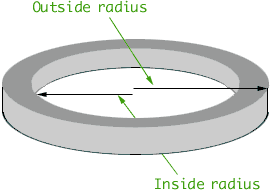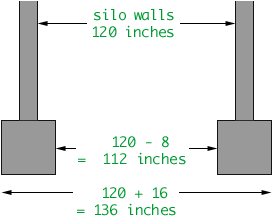Name: Natasha Who is asking: Student Level: Secondary Question: We wish to build a circular silo with internal diameter 10 feet. How much concrete will we need to pour the foundation, if we only need a 1 foot wide and 1 foot deep ring on which the silo walls will sit? Assume the 4 inch thick silo wall rests on the middle of the ring. Hi Natasha, The volume of concrete is the difference of the volumes of two circular cylinders. The first has radius the "outside radius" in the diagram below and the second has radius the "inside radius". I would measure each radius in inches.The volume of a cricular cylinder is pi x radius2 x height. Both cylinders have height 12 inches so the problem can be solved if you find the radii. To determine the inside and outside radiud draw a cross-section of the silo, through a diameter.You can use this information to find the volume of concrete needed in cubic inches. When you purchase concrete, the price is quoted in cubic yards. (The contractors actually call it "yards".) You should probably report your answer in cubic yards. Cheers, Penny Go to Math Central